## Abhiroop Sarkar

### OUTLINE

• Motivation
• My Master's Thesis
• Lift-Vector : A library for vector programming
• An alternate programming model?
• Automatic Vectorization (if time permits)

## Types of Parallel Machines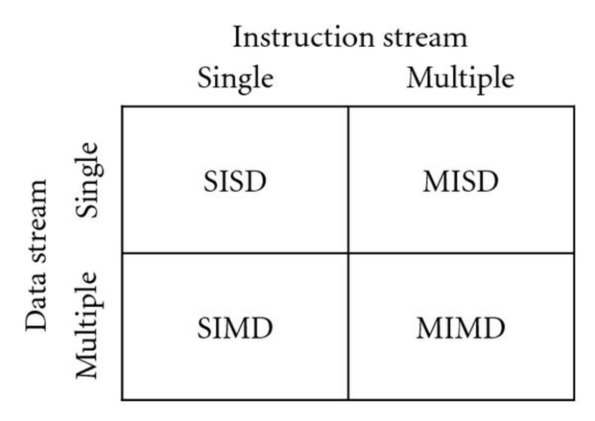### Single Instruction Multiple Data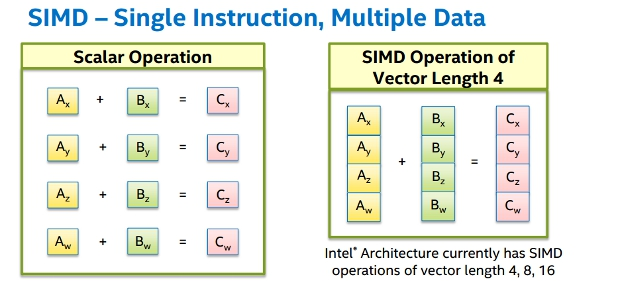## WHY SIMD?

• Power efficient
• Relative low cost in die area
• Lesser FDE cycles for a given data size
• ML algorithms are data parallel in nature
• Numerical computing algorithms are data parallel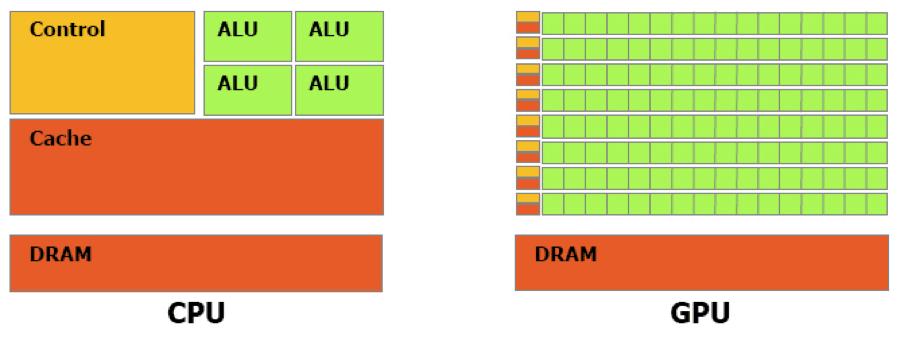We focus on CPU vector programming.

• More flexible memory model compared to GPUs
• Easier to implement stack and recursion in CPUs
• GPU data transfer bandwidth capped at 15 GB/s

## x86 Instruction Set

• Intel
• SSE (Intel P6, 1999)
• SSE2 (Intel Pentium M, 2003)
• SSE3 (NetBurst - Pentium 4, 2004)
• SSE4.1 (Intel Core, 2006)
• SSE4.2 (Nehalem, 2008)
• AVX (SandyBridge, 2011)
• AVX2 (Haswell, 2013)
• AVX512 (Canon Lake, 2018)
• AMD

## Compiler Support

• GCC
• LLVM Toolchain
• Dyalog APL

## Software Support

• OpenMP
• OpenCL

Can a purely functional language support vectorisation?

Enriching the question...

Can a purely functional language support vectorisation, preserving its original syntax and semantics while being as efficient as low level systems programming languages?

We will attempt to answer this throughout the rest of the presentation

## MASTER'S THESIS

### Superword Level Parallelism in the Glasgow Haskell Compiler

We will avoid talking about the engineering aspects and try to focus on the research side of the problem.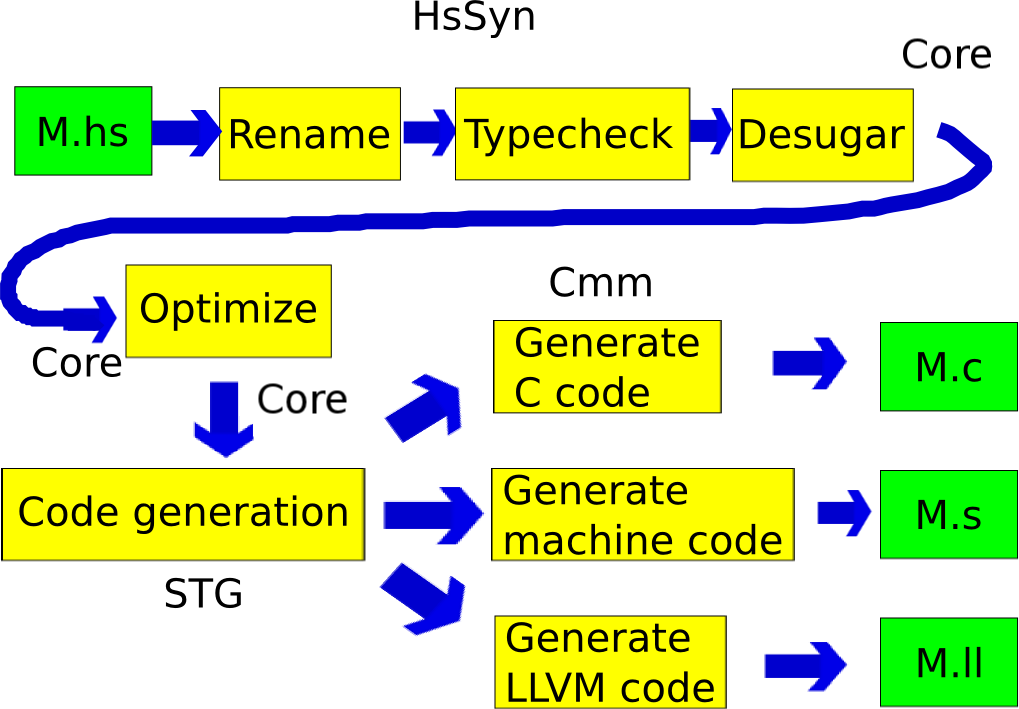`Float` supports `FloatX4`, a vector data type

`Double` supports `DoubleX2`, a vector data type

The user-level API

`````` -- constructors
packFloatX4#      :: (# Float#, Float#, Float#, Float# #) -> FloatX4#
-- destructors
unpackFloatX4# :: FloatX4# (# Float#, Float#, Float#, Float# #)
-- operations
plusFloatX4#   :: FloatX4# -> FloatX4# -> FloatX4#
minusFloatX4#  :: FloatX4# -> FloatX4# -> FloatX4#
timesFloatX4#  :: FloatX4# -> FloatX4# -> FloatX4#
divideFloatX4# :: FloatX4# -> FloatX4# -> FloatX4#``````

SSE as well as AVX support

Example : Vectorizing dot product

This spoils the declarative nature of Haskell!

# Lift-Vector

Polymorphic SIMD functions for vector programming

Lift-vector provides two interfaces

• Data.Primitive
• Data.Operations

Data.Primitive

``````class (Num v, Real (Elem v)) => SIMDVector v where
type Elem v

type ElemTuple v

nullVector       :: v
vectorSize       :: v -> Int
elementSize      :: v -> Int
broadcastVector  :: Elem v -> v
mapVector        :: (Elem v -> Elem v) -> v -> v
zipVector        :: (Elem v -> Elem v -> Elem v) -> v -> v -> v
foldVector       :: (Elem v -> Elem v -> Elem v) -> v -> Elem v
sumVector        :: v -> Elem v
packVector       :: ElemTuple v -> v
unpackVector     :: v -> ElemTuple v``````

Data.Operations

``````class (Num a, Num b) =>
ArithVector t a b
where
-- | The folding function should be commutative.
fold :: (a -> a -> a) -> (b -> b -> b) -> b -> t b -> b
zipVec ::
(a -> a -> a) -> (b -> b -> b) -> t b -> t b -> t b
fmap  :: (a -> a) -> (b -> b) -> t b -> t b
-- | Work efficient Blelloch Scan
scanb :: (a -> a -> a) -> (b -> b -> b) -> b -> t b -> t b``````

## Vectorised Containers

• Vector Lists
• Vector Arrays (Shape polymorphic)

### Vector Lists

``newtype VecList a = VecList [a]``

A wrapper over plain lists

### Vector Arrays

Wrappers around unboxed vectors.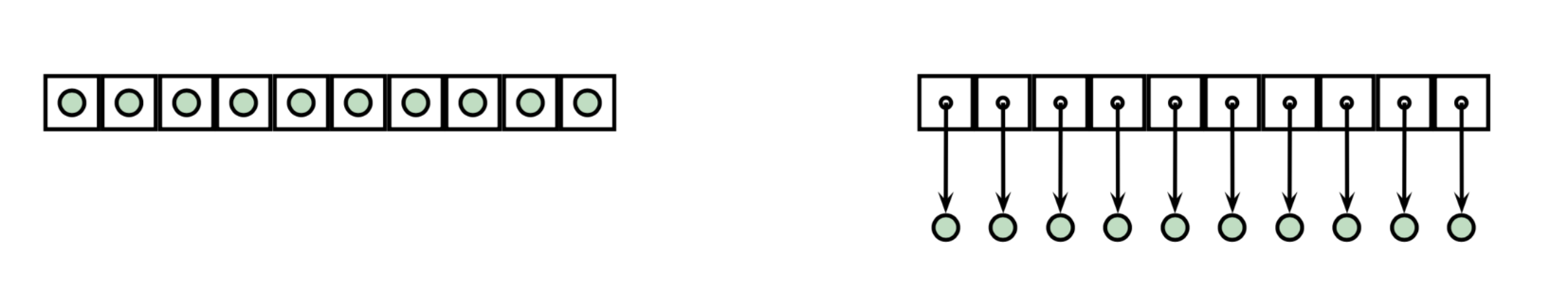``````
import qualified Data.Vector.Unboxed as U

data VecArray sh a = VecArray !sh (U.Vector a)``````

What does dot product look like with the parallel operators?

Vector Lists

``````dotVec :: [Float] -> [Float] -> Float
dotVec xs ys =
fold (\x y -> x + y :: FloatX4) (\x y -> x + y :: Float) 0 \$
zipVec
(\x y -> x * y :: FloatX4)
(\x y -> x * y :: Float)
(toVecList xs)
(toVecList ys)``````

Vector Arrays

``````dotVec' :: [Float] -> [Float] -> Float
dotVec' xs ys
= let l1 = length xs
l2 = length ys
in fold (\x y -> x + y :: FloatX4) (\x y -> x + y :: Float) 0 \$
zipVec
(\x y -> x * y :: FloatX4)
(\x y -> x * y :: Float)
(toVecArray (Z :. l1) xs)
(toVecArray (Z :. l2) ys)``````

How are the `Data.Operations` operators implemented?

### ZIP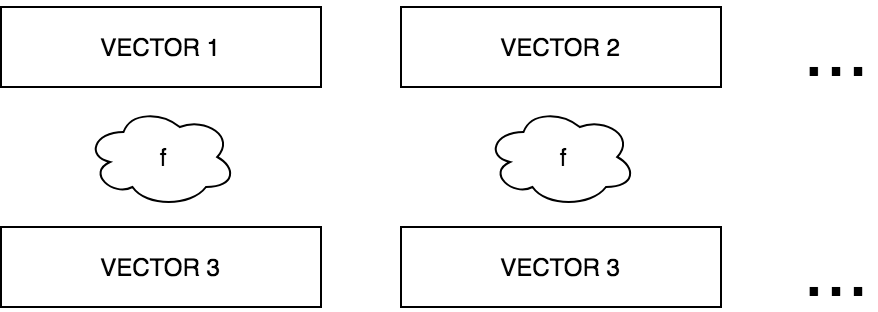### MAP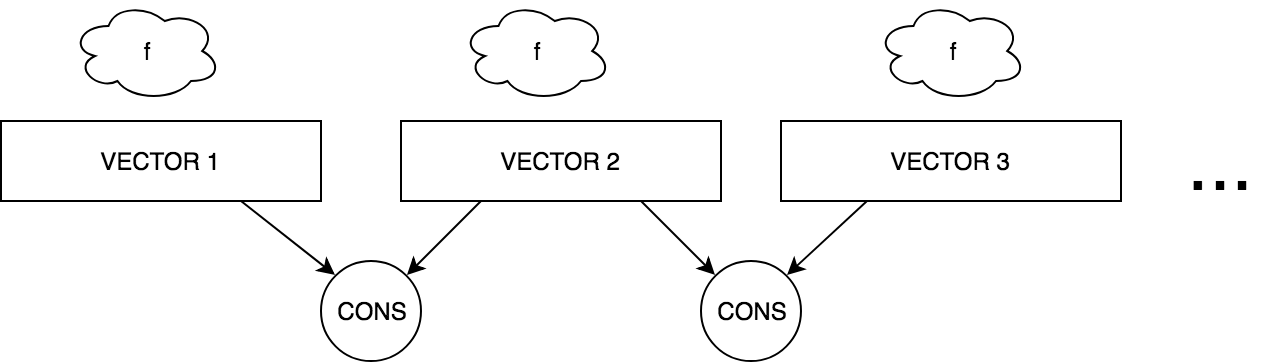### FOLD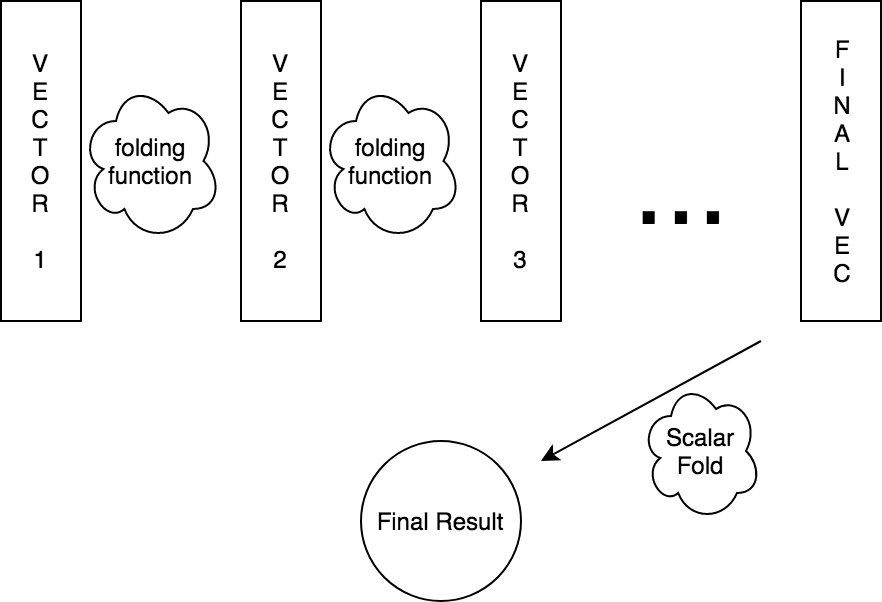### SCAN

`scan` or prefix sum looks like an inherently sequential operation

``````> scanl (+) 0 [1, 2, 3, 4, 5, 6, 7, 8]
> [0,1,3,6,10,15,21,28,36]``````

Lift-vector supports a work efficient parallel scan

## BLELLOCH SCAN

### UPSWEEP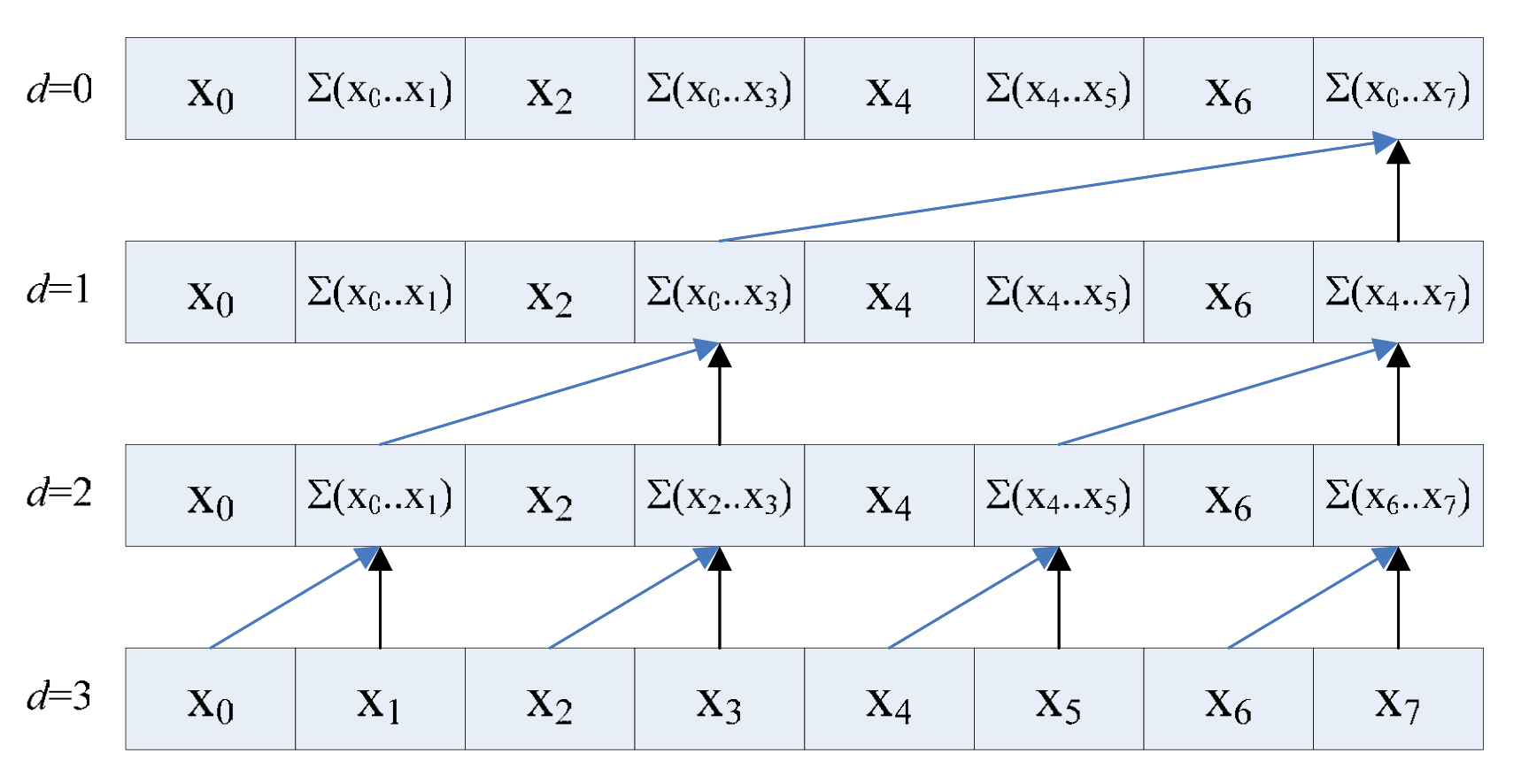### DOWNSWEEP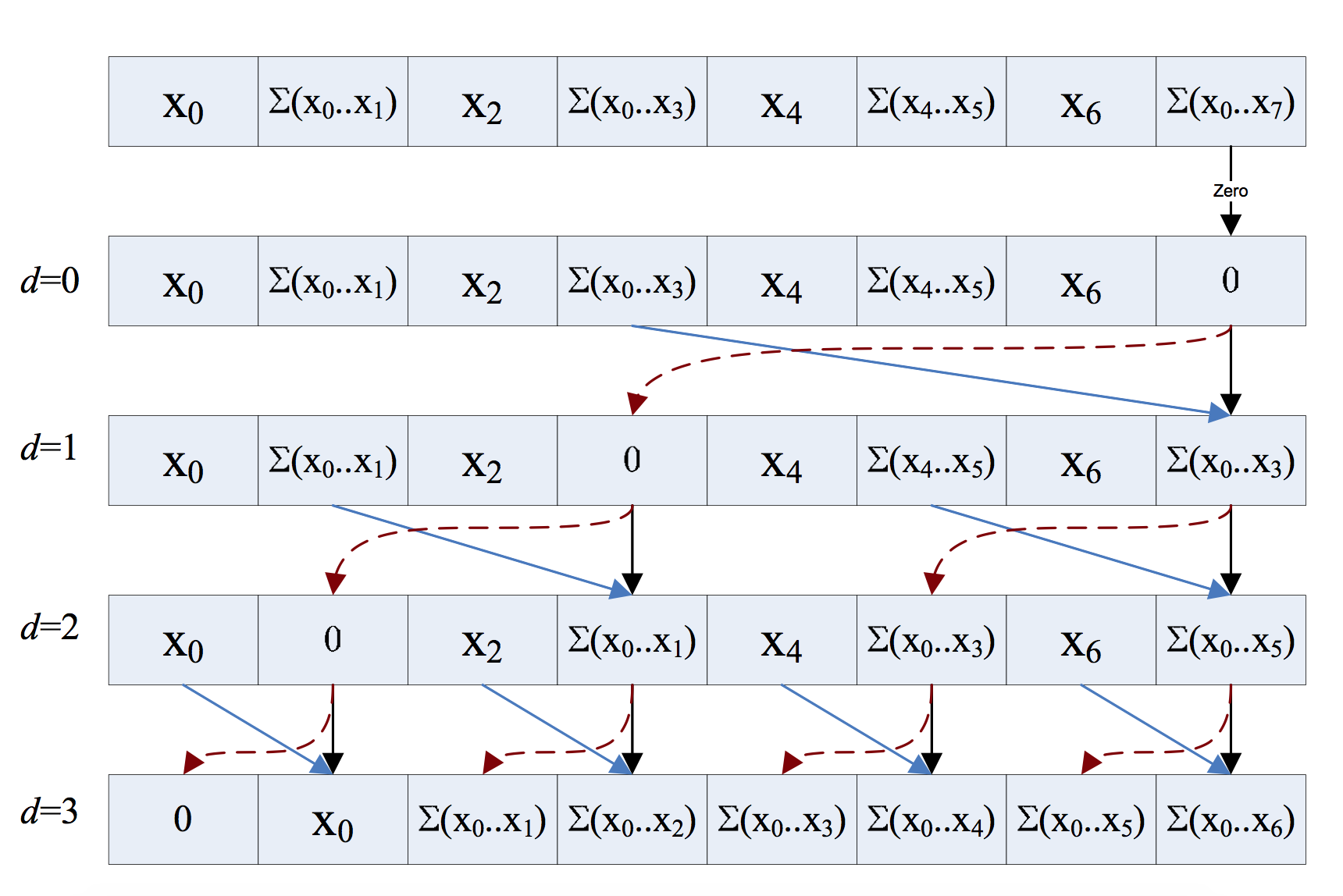Benchmarks

Good benchmarks

Pearson Correlation Coefficient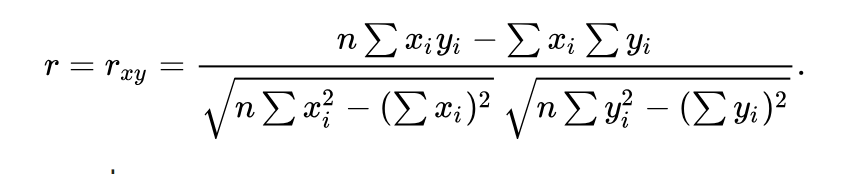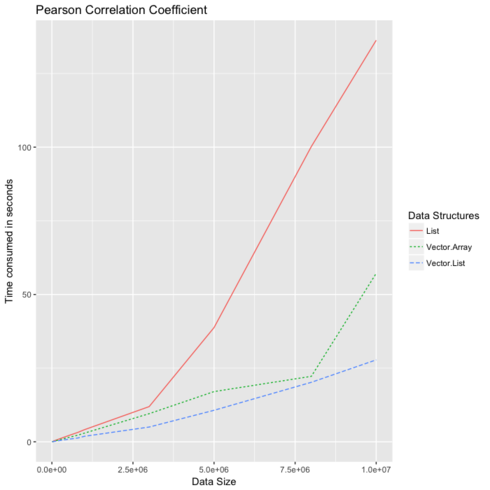Heavy writes

Eg: Matrix Multiplication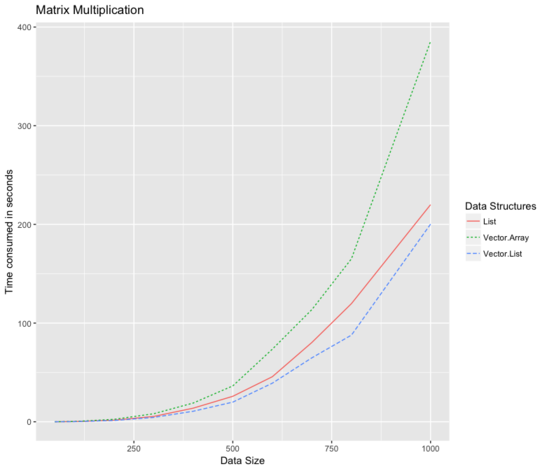## Lift Vector

https://github.com/Abhiroop/lift-vector

• Leaky abstraction
• Supports just flat and regular data parallelism
• No focus on optimizing memory layout
• Number of rewrite opportunities missed
• ...

### EXAMPLE

``````import qualified Data.Operations as Op

foo :: Float -> Float
foo x = x*x + 1

foo' :: [Float] -> [Float]
foo' xs =
Op.fromVecList \$
Op.fmap (\x -> x + 1 :: Float) (\x -> x + 1 :: FloatX4) \$
Op.fmap (\x -> x * x :: Float) (\x -> x * x :: FloatX4) \$
Op.toVecList xs``````

Any calls to `map foo` should be replaced by `foo'`

Now,

``````bar :: [Float] -> [Float]
bar xs = map foo xs

bar :: [Float] -> [Float]
bar = foo'

bar' :: [[Float]] -> [[Float]]
bar' xss = ???``````

### Blelloch's solution

Flatten all levels of nesting

``````concat   :: (ArithVector t) => t (t a) -> t a
unconcat :: (ArithVector t) => t (t a) -> t b -> t (t b)

bar' :: [[Float]] -> [[Float]]
bar' xss = unconcat xss (bar (concat xss))``````

This is manually impossible to define in a library

Need the compiler to understand nested structures

### MEMORY LAYOUT ISSUES

Records are desugared to Product Types.

Very common to have array of tuples.

`VecArray (Float, Float, Float)` or `VecList (Float, Float, Float)`

Current hardware supports load and store vector instructions only for contiguous data

*Xeon Phi instruction set supports non contiguous loads and stores

Generally structure of arrays (SOA) layout is optimal over array of structures (AOS) layout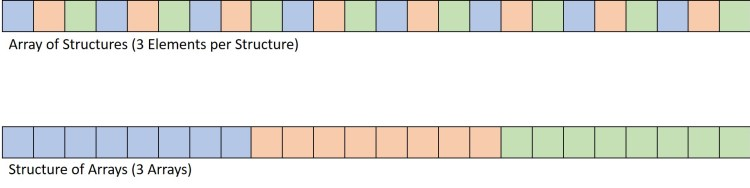This was not explored in the existing design of lift-vector

What are we looking for?

• An alternate programming model for programming vectors
• A smart compiler (automatic vectorization)

Programming model

• Data Layout
• Syntax
• Semantics
• Data layout
• SOA over AOS
• Flatten nested parallelism
• Handle nested iterators (Palmer et al. 1995)
• ... more exploration
• Semantics of the model
• Parallel by default
• Express divergent control flow (Karrenberg et al. 2017)
• Differentiate between convergent and divergent control flow
• Eagerly evaluated
• Leverage fusion wherever possible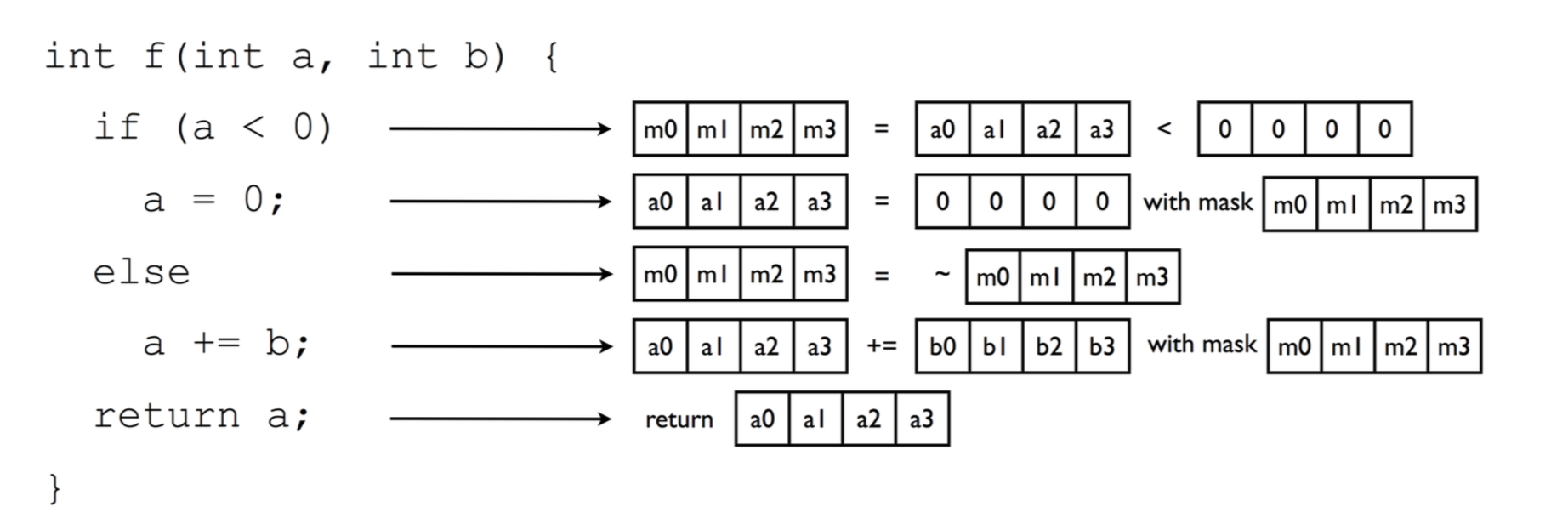Whole function vectorization : control flow to data flow - Karrenberg, 2017

• Syntax
• Declarative

### Example

`````` -- all functions have parallel semantics unless specified otherwise
quicksort :: [Float] -> [Float]
quicksort [] = []
quicksort xs =
let pivot = srandom xs -- assume srandom pure & sequential
small = select (p <= pivot) xs
large = select (p >  pivot) xs
in (quicksort small) ++ [x] ++ (quicksort large)
gang1                         gang2
-- irregular data size but NDP can be made work efficient``````

Simply uses relational operators

Vector instruction `CMPPS`in SSE or `VCMPPS` in AVX

Merge sort is easier to parallelize

`````` [16 elems]    [16elems]
8      8      8     8
4 4    4 4    4 4   4 4
mergesort_spmd xs
let pivot    = smiddle xs
(x1, x2) = split xs
in merge (mergesort_spmd x1) (mergesort_spmd x2)

merge :: [a] -> [a] -> [a] - Vectorized SIMD union``````
``````            xs = [9,7,8,1]
/    |     \    \
/     |      \    \
/      |       \    \
/       |        \    \
(fork xs) (fork xs) (fork xs) (fork xs)

foo = pack (9,7,8,1)
(a,b)    foo < bar``````

An existing model on GPUs - SPMD (OpenCL)!

Intel ispc compiler - SPMD on SIMD

## Why not just use ISPC?

• No nested data parallelism
• Impure, doesn't provide "safe" parallelism
• A systems language replicating the entire C pointer model
• Explicit uniform and varying memory annotations

So how do we implement our language?

### Engineering decision

• GHC might be coaxed to admit nested data parallelism (using source plugins) but SPMD requires major changes in the runtime.
• Write a small functional frontend to LLVM which flattens the data as an optimizer pass.
• Cajole the NESL runtime to an SPMD form and connect it to LLVM.

## Conclusion

Haskell has shown that performance at the cost of expressivity is not necessary

There is a always a sweet spot!

## Reference

 Exploiting Vector Instructions with Generalized Stream Fusion. Mainland et al

THANK YOU FOR LISTENING

EXTRA SLIDES

### DISADVANTAGES OF THE SPMD-NDP MODEL

• complex control flow
• V.IMP: Full vectorization can be disastrous
• Flattening destroys the original loops and spoils a number of optimization opportunities

### COST OF VECTORIZATION

• Cost model
• Work and Depth (Blelloch, 1996)
• Compiler cost model (reducing pack, unpacks, scatters, gathers)

### Throttled Superword Level Parallelism

Convert IR to SSA form

Cytron's algorithm

Hand made graph still SLP vectorized version slower than sequential version of the program

Throttle the vectorization by cutting the graph

Throttling is NP Hard

Can we auto vectorise faster?